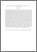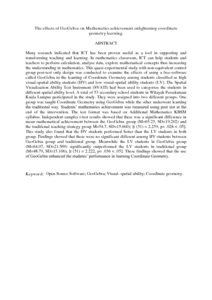# The effects of GeoGebra on Mathematics achievement enlightening coordinate geometry learning.

## Citation

Abdul Saha, Royati and Mohd. Ayub, Ahmad Fauzi and Ahmad Tarmizi, Rohani (2010) The effects of GeoGebra on Mathematics achievement enlightening coordinate geometry learning. Procedia - Social and Behavioral Sciences, 8 (2010). pp. 686-693. ISSN 1877-0428

## Abstract

Many research indicated that ICT has been proven useful as a tool in supporting and transforming teaching and learning. In mathematics classroom, ICT can help students and teachers to perform calculation, analyse data, explore mathematical concepts thus increasing the understanding in mathematics. This quasi-experimental study with non-equivalent control group post-test only design was conducted to examine the effects of using a free-software called GeoGebra in the learning of Coordinate Geometry among students classified as high visual-spatial ability students (HV) and low visual-spatial ability students (LV). The Spatial Visualization Ability Test Instrument (SVATI) had been used to categorize the students in different spatial ability level. A total of 53 secondary school students in Wilayah Persekutuan Kuala Lumpur participated in the study. They were assigned into two different groups. One group was taught Coordinate Geometry using GeoGebra while the other underwent learning the traditional way. Students’ mathematics achievement was measured using post test at the end of the intervention. The test format was based on Additional Mathematics KBSM syllabus. Independent samples t-test results showed that there was a significant difference in mean mathematical achievement between the GeoGebra group (M=65.23, SD=19.202) and the traditional teaching strategy group M=54.7, SD=15.660); [t (51) = 2.259, p= .028 < .05]. This study also found that the HV students performed better than the LV students in both group. Findings showed that there were no significant different among HV students between GeoGebra group and traditional group. Meanwhile the LV students in GeoGebra group (M=64.07, SD=21.569) significantly outperformed the LV students in traditional group (M=48.79, SD=15.106); [t (51) = 2.222, p= .036 < .05]. These findings showed that the use of GeoGebra enhanced the students’ performance in learning Coordinate Geometry.Preview
PDF (Abstract)
The effects of GeoGebra on Mathematics achievement enlightening coordinate geometry learning.pdfView Item